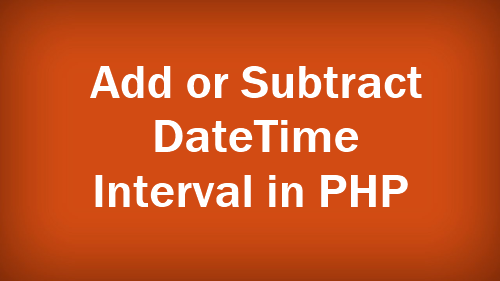# How to Add or Subtract Date Interval In PHPAdding or subtracting interval to a DateTime instance is quite easy. Two methods we are going to described in this post. Both methods belong to DateTime object itself.

If you are using PHP 5.3+ version, than simply add and subtract method work for it.

See below example to Add 2 Day interval in date.

PID use for 1 day time interval, If you need to add 2 days, 1 month and 4 years than use P4Y1M2D.  To include time period interval format like that P1DT2H. It will add 1 day and 2 hours interval in date time.

To Subtract interval in DateTime check below example:

For PHP 5.2 version simple modify method work for date interval. To add three days interval, just use ‘+3 day’. Check example code:

So use these simple method to Add or Subtract date time interval. In our previously blog post described Changing Date and Time Format. Share this post with others. Thanks